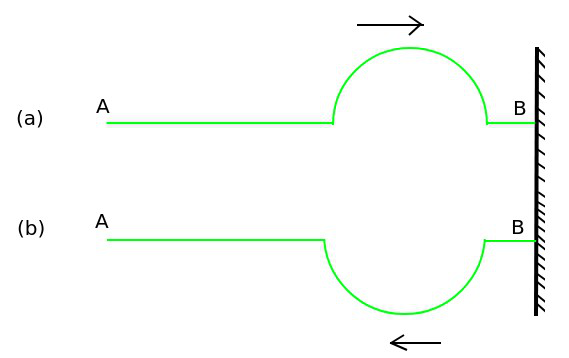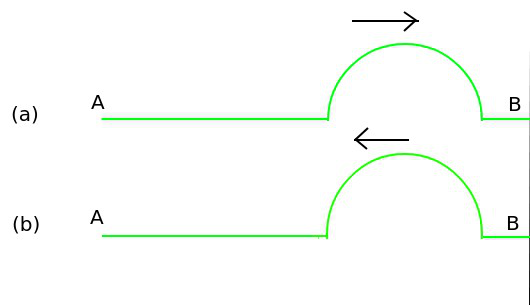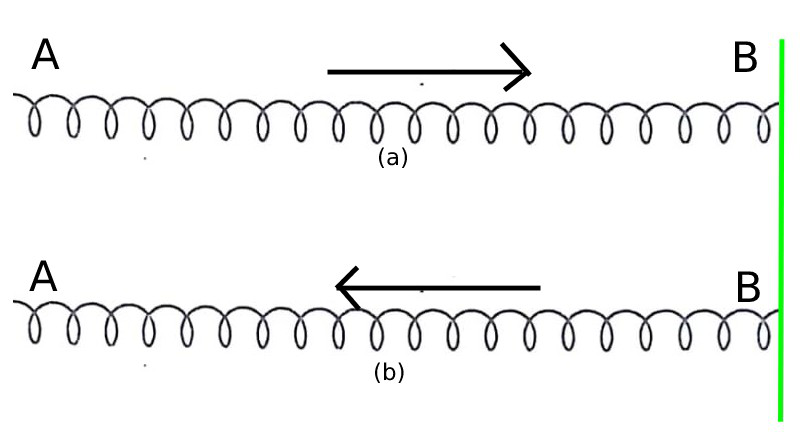GFG App
Open AppBrowser
Continue

Waves are an integral part of our earth and nature. Waves are found in nature in many forms such as sound waves, light waves, water waves, radioactive waves, ultraviolet waves, and mechanical waves like transverse waves and longitudinal waves. Energy is transferred through waves. Due to its free movement in nature, many types of properties are found in waves.

Events like a reflection of waves, superposition of waves, displacement of waves, acceleration of waves, etc. A wave is a type of oscillation (disturbance) that flows over time and space. Wave movements move energy from one location to another. Waves play a significant part in our daily lives. This is due to the fact that waves can transport energy and information across long distances. Waves need an oscillating or vibrating source. The first known waves were ocean surface waves. Later, more types were discovered.

So in this article, we are going to learn about the reflection of waves, we will learn how many types of wale reflections are present in nature. how humans use the properties of wave reflection in their daily life.

### Reflection of Waves

When a progressive wave hits two boundary surfaces of different densities, it returns to the same medium following certain laws. This phenomenon is called Reflection of the Waves

In the phenomenon of reflection, progressive waves obey the same laws as light waves. The reflection of a progressive wave from a boundary surface depends on the nature of the boundary surface. Depending on the nature of the progressive wave, the reflection at the boundary surface can be of the following two types:

1. Reflection on the rigid wall, and
2. Reflection at the free end.

When a progressive wave traveling through a rarer medium is reflected from a denser medium, it is called Reflection at the hard wall, and when a progressive wave traveling through a denser medium is reflected from a rarer medium, it is called Reflection at the free end.

### Reflection of a Transverse Pulse from a Rigid Boundary

Suppose the end B of a string AB is tied to a rigid base, a wave pulse is sent from its end A from left to right. If the displacement of the particles in this pulse is upward. When this pulse reached a firm base, it exerts a force on the base in an upward direction. Consequently, according to Newton’s third low motion, a firm base that exerts the same reaction force produces a pulse that starts moving in the opposite direction (from right to left) with the help of a string. This is reflected pulse.In the incident pulse and the reflected pulse, the particles of the string vibrate in opposite directions. Thus, when a pulse is reflected from a rigid boundary, it gets inverted with respect to the incident pulse.  That is, as a result of reflection from the rigid boundary, there is a change of pi or 180° in the phase of the incident pulse.

If the equation of the incident wave is,

y = A sin 2π / λ (vt – x)

Then the equation of the reflected wave will be,

y = A sin 2π / λ (vt + x + pi)

or

y = – A sin 2π / λ (vt + x)                                                         (because sin( θ – π ) = -sin θ)                                  …… (1)

Thus, if the equation of the incident wave is,

y = A sin(ωt – kx)

Then the equation of the reflected wave will be,

y = -A (ωt + kx)                                                                                                                                                       …… (2)

It is assumed that the incident wave is traveling in the positive direction along the X-axis and the reflected wave is traveling in the negative direction along the x-axis. When a progressive wave traveling from a denser medium gets reflected in a rarer medium, it is called Reflection at the free boundary.

### Reflection of a Transverse Pulse from a Free Boundary

Suppose the end B of the string AB is attached to a small ring which can make frictionless oscillations in a vertical rod. A wave pulse is sent from left to right in the string. Suppose the displacement of the particles of the string is upwards. When this pulse reaches end B, it exerts an upward force on this end B. Hence the ring attached to end B moves upwards.

While returning, this ring crosses the equilibrium position due to inertia. In this case, the ring exerts a reaction force on the string, due to which the same type of pulse starts moving in the opposite direction in the string. Thus, in the incident pulse and the reflected pulse, the particles of the string vibrate in the same direction. It is clear that there is no change in the phase of the incident pulse as a result of reflection from the free boundary.Thus, if the equation of the incident wave is,

y = A sin 2π / λ (vt – x)

Then the equation of the reflected wave will be,

y = A sin 2π / λ (vt + x)                                                   (because sin(θ – π) = -sin θ)                                                                   …… (3)

Thus, if the equation of the incident wave is,

y = A sin(ωt – kx)

Then the equation of the reflected wave will be,

y = A (ωt + kx)                                                                                                                                                                               …… (4)

### Reflection of the Longitudinal Pulse from Rigid Boundary

If end B of spring AB is tied to a rigid base. With the help of this spring, a compression pulse is sent from left to right (from A to B). When the pulse reaches end B, it exerts a force on a rigid base from left to right. According to Newton’s third law of motion, a rigid base exerts an equal reaction force on the spring end B from right to left.

As a result, the compression pulse starts moving from end B to end A with the help of spring. In the incident pulse and the reflected pulse, the directions of displacement of the spring particles are opposite. Therefore, in case of reflection from a rigid base, the phase of the incident pulse changes by π or 180°.In this situation also, the reflected wave can be represented by equation (1) or (2).

### Reflection of the Longitudinal Pulse from the Free Boundary

Let AB be a spring. A compression pulse moves from end A to B i.e. from left to right with the help of spring. When it reaches the free end B, the pulse exerts a force on B from left to right. Due to this force, end B crosses the equilibrium position and moves to the right. Hence, the length of the spring increases, and the rarefaction pulse starts moving from right to left, but still, the direction of displacement of the spring particles remains the same. Therefore, there is no change in its phase due to the reflection of longitudinal pulse from the free boundary.

In this situation also, the reflected wave can be represented by equation (3) or (4).

### Application of Reflection of Waves

• Echo: If we utter some words standing at some distance near a hill, tall building, big tree, etc., then due to reflection, the same type of words are heard again. This phenomenon is called Resonance. The phenomenon of repetition of sound due to the reflection of sound from a rigid boundary is called echo.
• Due to the Persistence of hearing, the effect of sound remains on our ear for 0.1 seconds. Therefore, to hear the echo separately from the original sound, it is necessary that the reflected sound should reach our ear after 0.1 seconds after hearing the original sound. For this, the reflecting surface needs to be at a reasonable distance from the listener. Therefore, to hear the echo clearly, the minimum distance of the listener from the reflecting surface should be 17 meters. The reflection of sound is used in measuring the depth of the sea or lake, soundboard, megaphone, etc.
• Sonar: SONAR Its full name is “Sound Navigation and Ranging”. It is based on the principle of reflection of ultrasonic waves. With its help, the position of anybody inside the sea such as a rock, enemy submarine, etc. is detected.  The depth of the sea is also known by this technique.

### Sample Problems

Problem 1: Name the good and bad reflector or sound waves and also give the name of substance which is an absorber of sound?

Solution:

Following are the good, bad, and good absorbers of sound waves:

1. Hard objects are a good reflection of sound because particles bounce straight off them. For example – Tiles and Marble slabs.
2. Bad reflectors are those objects which absorb sound. For example –  Thick curtains and thick carpets.
3. Objects that absorb the sound well are called good absorbers of sound. For example – Holed cardboard.

Problem 2: A radar sends a signal to an aircraft at a distance of 70 km away and receives it back after 3×10-2 seconds. What is the speed of the signal?

Solution:

Given that,

Distance of aircraft = 70 km = 70,000 m

The Total distance = 3 × 70,000 = 2,10,000 m

The Time taken = 3 × 10-2 seconds

Hence Speed of signal (V) = Total distance traveled (2d) ​/ time taken (t)

= 2,10,000 / 3×10−2

= 7 × 106 m/s

Problem 3: Mention any two applications of reflection of sound.

Solution:

Following are the applications of reflection of sound:

1. Hearing aid: A gadget used by persons who are deafeningly deafeningly deafeningly deafeningly deafeningly deaf The sound waves emitted by the hearing aid are reflected into a smaller region heading to the ear.
2. Soundboards: Curved surfaces have the ability to reflect sound waves. This sound wave reflection is utilized in auditoriums to disperse the waves evenly across the venue. Sound Boards are used to reflect sound waves. The speaker is situated in the center of the soundboard.

Problem 4: Light in the air has a frequency of 6 ×1012 ​Hz. What is the frequency of light when it penetrates water?

Solution:

Only the velocity and wavelength of light change during refraction. When light enters from air or vacuum, i.e. when light moves from one medium to another, its frequency does not change, i.e. it remains constant.

As a result, the frequency of light will remain 6 ×1012 ​Hz.

Problem 5: Define the phenomenon of Resonance.

Solution:

Resonance is a phenomena in which the inherent frequency of a body equals the frequency of an external periodic force and the amplitude of vibrations becomes very big.

My Personal Notes arrow_drop_up